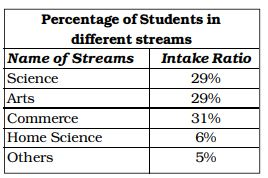## Introduction to Data Interpretation

#### Data Interpretation

Direction: The following table gives the result of a survey based on newspaper reading habits. Study the table and answer the questions.1. The number of people who read only English newspapers.

1. According to given table, we have
Number of people who read only English Newspapers = 123 + 206 + 325 = 654

##### Correct Option: B

According to given table, we have
Number of people who read only English Newspapers = 123 + 206 + 325 = 654
Therefore , option B is correct answer .

Direction: The table given below shows production of five types of cars by a company from the year 1998 to 2003. Study the table and answer the question.1. In which year the production of cars of all types taken together was approximately equal to the average of the total production during the period:

1. On the basis of given table in question ,
Total production of cars of all types taken together = 476
Number of given years = 6

 Average production of whole duration = Total production of cars of all types taken together Number of given years

##### Correct Option: B

On the basis of given table in question ,
Total production of cars of all types taken together = 476
Number of given years = 6

 Average production of whole duration = Total production of cars of all types taken together Number of given years

 Average production of whole duration = 476 = 79 6

which is total production in 2000.

1. Different choices made by a group of 200 students are given below in percentage. The number of students who have taken neither Science nor Commerce is1. According to given table, we have
Percentage of students in ( Science + Commerce ) = (29 + 31)% = 60%
Percentage of students in ( Arts + Home science + Others ) = (29 + 6 + 5)% = 40%
Percentage of students in all streams = 100%

##### Correct Option: A

According to given table, we have
Percentage of students in ( Science + Commerce ) = (29 + 31)% = 60%
Percentage of students in ( Arts + Home science + Others ) = (29 + 6 + 5)% = 40%
Percentage of students in all streams = 100%

 Students who neither have commerce nor science = 200 × 40 = 80 100

Direction: The following table shows the production of food grains (in million tonnes) in a State for the period 1988 to 1992. Read the table and answer the following questions.1. During 1992, the percentage of increase in the production of wheat, over the previous year was:

1. On the basis of given table in question ,
Production of wheat in 1992 = 860 million tonnes
Production of wheat in 1991 = 680 million tonnes
Increase in production = 860 - 680 = 180 million tonnes

 Percentage increase = Increase in production × 100 Production of wheat in 1991

##### Correct Option: A

On the basis of given table in question ,
Production of wheat in 1992 = 860 million tonnes
Production of wheat in 1991 = 680 million tonnes
Increase in production = 860 - 680 = 180 million tonnes

 Percentage increase = Increase in production × 100 Production of wheat in 1991

 Percentage increase = 180 × 100 = 26.47% 680

1. The increase in the production of other cereals (over the previous year) was minimum during the year :

1. As per the given above table , we see
Production of other cereals in 1988 = 350 million tonnes
Production of other cereals in 1989 = 400 million tonnes
Increase in production = 400 - 350 = 50 million tonnes

 Percentage increase in 1988 = 50 × 100 = 14.28% 350

Similarly , we can find other values .

##### Correct Option: A

As per the given above table , we see
Production of other cereals in 1988 = 350 million tonnes
Production of other cereals in 1989 = 400 million tonnes
Increase in production = 400 - 350 = 50 million tonnes

 Percentage increase in 1988 = 50 × 100 = 14.28% 350

Production of other cereals in 1989 = 400 million tonnes
Production of other cereals in 1990 = 420 million tonnes
Increase in production = 420 - 400 = 20 million tonnes
 Percentage increase in 1989 = 20 × 100 = 5% 400

Hence , required answer is 1989 year .